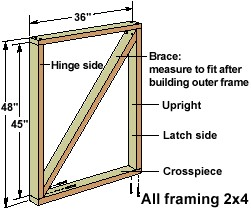# What Is The Importance Of Triangles In Our Daily Life?

Triangles feature in a number of aspects of our daily lives, including mathematics, engineering, architecture, astronomy, and even music.

• Triangles in mathematics

Triangles feature very frequently in the discipline of mathematics. For example, almost all two dimensional shapes (apart from a circle) can be made up of a series of triangles arranged in a certain way. Furthermore, in the field of mathematics, triangle-related formulae such as Pythagoras' theorem (a^2+b^2=c^2) form the basis of a great deal higher levels of mathematical education, in branches such as trigonometry.

• Triangles in engineering and architecture

As mentioned above, Pythagoras' theorem is an incredibly important theory. However, its importance goes beyond the field of pure mathematics and spans other fields including engineering and architecture. Disciplines such as these, which focus a great deal upon the safe distribution of weight or force, for example, rely heavily on Pythagoras' theorem, which is entirely concerned with triangles.

Furthermore, many bridges and other similar structures are often designed to include triangle shapes, as these shapes are able to withstand a great amount of pressure (in a similar way to arches). Because of the way that triangles disperse pressure throughout their shape, they are able to withstand more pressure than a differently-shaped object (for example, a square) of the same size.

• Triangles in astronomy

Principles of trigonometry, or the study of triangles, are used widely in fields such as astronomy, space travel and communication in ways that I, as a non-astronomer, cannot even begin to understand. However, my research suggests that trigonometry plays a role in aspects of astronomy such as deciding how far about the earth a satellite dish should be placed.

• Triangles in music

Unfortunately, as with the field of astronomy, I cannot begin to understand how trigonometry is featured in music, nor could I explain this to you. However, as a rough guide, trigonometry features in music theory, and can explain some questions surrounding the production of sound itself.
thanked the writer.
Surveyors use them to calculate the height of trees/ buildings
thanked the writer.
It has the following uses
If you put three points anywhere in space (not in a straight line) you have exactly one plane that goes through all three. This is unique to three points, any more points and there may not be a plane and any less and there are multiple planes. This is useful as it means that a three-legged stool will be stable no matter how uneven the ground7. It also helps simplify some calculations, for example, those in 3-D graphics.

In 3-D graphics, the surfaces of 3-D objects are broken down into triangles. Small numbers of triangles are used for flat surfaces, while large numbers are used to mould curved surfaces similar to the way a geodesic dome is constructed8. The triangle vertices are computed on an X-Y-Z scale and every one must be recomputed each time the object is moved.

Triangluation is using the trigonometry of sines cosines and Pythagoras's Theorem to find your location assuming you know two angles and a length of one side a triangle. Trilateration is how to work out your exact point in space assuming you know the distance to three (if you are working on a plane) or four (in 3D) points. Triangulation and trilateration are used for many purposes, including surveying, navigation, metrology (the science that deals with measurement), astrometry (the measurement of the positions and motions of celestial bodies), binocular vision and gun direction of weapons. They are the basis on which GPS (Global Positioning System) works.
Triangles have many uses in construction due to their strong and rigid shape. They are the basis of many buildings including bridges, monuments9 and domes. The geodesic dome is one of the most stable of geometric forms and is made of many triangles (as tetrahedrons) which distribute stress evenly to all elements of the dome, providing a high strength-to-weight ratio. The dome of the Eden Project is an example of such a geodesic dome, although this dome is of triangles arranged together as hexagons and pentagons.

1 Euclidean geometry is the familiar kind of geometry on the plane or in three dimensions.
2 While this certainly appears to be true, it can't actually be proved, and non-Euclidean geometries in which the angles add up to less than or more than 180° have been found to be completely consistent, although not bearing much relevance to Planet Earth. They imply the existence of curved space.
3 Also sometimes known as a 'right triangle'.
4 Plural: Vertices.
5 Perpendicular means forming a right angle with.
6 This is also known in common language as the height.
7 As long as the ground is not so uneven that the stool actually falls over.
8 It takes an enormous number of triangles to simulate totally round objects.
9 For example: The Eiffel Tower, The Statue of Liberty and the Crystal Palace.
thanked the writer.
Triangle structures can be very strong, so are often used in the construction and fabrication trades. These are often seen in the form of diagonal braces.thanked the writer.
Making of electric transformers on bridge..
thanked the writer.
Used in measuring the height of towers...
thanked the writer.

i want some more examples to prove the use of triangles in our daily life

thanked the writer.
It is used to measure the length of objects in  real life

Anonymous
thanked the writer.